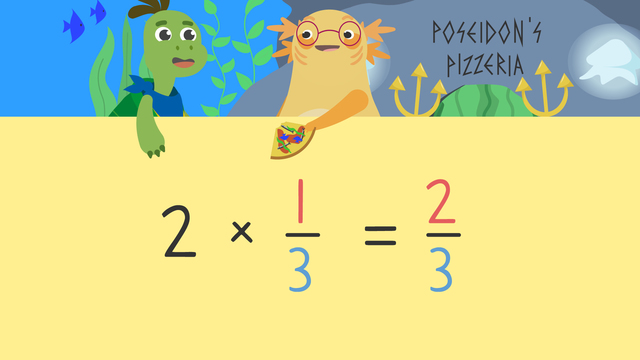# Fractions as a Multiple of Unit FractionsRate this video

Ø 5.0 / 1 ratings

The authorTeam Digital
Fractions as a Multiple of Unit Fractions
CCSS.MATH.CONTENT.4.NF.B.4.A

## Information about the videoFractions as a Multiple of Unit Fractions

### Contents

#### What is a Unit Fraction?

A unit fraction is one part of a whole that is divided into equal parts. A unit fraction has 1 as the top number, which is the numerator.

What is a unit fraction example? ⅙ is a unit fraction.

#### What is a Multiple of a Unit Fraction?

Multiples of unit fractions are fractions created by multiplying the fraction by whole numbers. Here are some examples:#### In this Video

Axel and Tank are out to dinner at their favorite pizza place. They need to figure out which pizza has enough slices to make a whole pizza for them to share. They will use multiples of unit fractions to determine the amount of pizza. We will learn what is a unit fraction 4th grade and how to make multiples of unit fractions.

#### Follow-Up Activities for Fractions as Multiples of Unit Fractions

After the video there is continued practice with learning ‘ What is a unit fraction in math?’ and ‘What is a multiple of a unit fraction?’ with multiples of unit fractions worksheets.

### TranscriptFractions as a Multiple of Unit Fractions

Axel and Tank are out to dinner at their favorite pizza place. They need to figure out which pizza has enough slices to make a whole pizza for them to share. We can help Axel and Tank determine how much of each pizza there is by calculating "Multiples of a Unit Fraction". A unit fraction is a fraction that has one in the numerator and a whole number in the denominator. One-sixth is an example of a unit fraction. The whole is divided into six equal parts... and each part has a value of one-sixth. We can use repeated addition to find multiples of unit fractions... or look at them as multiples on a number line. We can also solve for the multiple of a unit fraction by setting up a multiplication equation. Let’s look at the anchovy pizzas in the case. The anchovy pizzas are divided into thirds… and each slice has a value of one-third. Now, look at the number of pizza slices. There are TWO, "one-third slices". We can write this as a multiplication equation to calculate the total amount of anchovy pizza available. First, write the number of unit fractions being grouped. There are two. Next, write the unit fraction that is represented. The unit fraction is one-third. We are going to multiply two times one-third. First, let's look at the denominator. The whole is STILL divided into thirds, so the denominator in the answer doesn’t change. Now, multiply the whole number and the numerator … two times one is two. The anchovy pizza has two-thirds of a pizza available... which is not enough for Axel and Tank to share a whole pizza. [Disappointed let's out a big sigh] "Sigh!" Next let's calculate how much of the Veggie Delight pizza is available. First, look at the number of unit fractions being grouped... and the unit fraction. How many slices, or unit fractions, of pizza, are being grouped? (...) There are four. What is the unit fraction for the veggie pizza? (...) Since there are five parts or slices, in a whole pizza the unit fraction is one-fifth. The next step is to write a multiplication equation. What are we multiplying? (...) Four times one-fifth. When we multiply, the denominator remains the same because the pizza is still sliced in fifths. Multiply the whole number with the numerator... four times one is four. There are four-fifths of a Veggie Delight pizza in the case... which is STILL not enough to make a whole pizza to share. Finally, let's calculate how much of the Under the Sea Supreme pizza is available. What is the first step? (...) Count how many unit fractions there are. (...) There are six. What is the second step? (...) Determine the unit fraction for the number of parts the whole pizza is divided into. What is the unit fraction for the Under the Sea Supreme? (...) The unit fraction is one-fourth. What is the next step? (...) Set up the multiplication equation. What are we multiplying? (...) Six times one-fourth. How much of the pizza is in the case? (...) There are six-fourths of Under the Sea Supreme pizza. Six-fourths or one and one half. There is more than enough for the very hungry Axel and Tank to order a whole pizza! Remember,(...) we can find the multiples of a unit fraction through multiplication. First, find the number of unit fractions being grouped together… and then, write the unit fraction being represented. Multiply the whole number and the unit fraction. When multiplying, start with the denominator. The denominator doesn't change. Finally, multiply the whole number with the numerator. [Excited to eat his pizza!] “Mmmmm,(...) Under the Sea Supreme!” “My FAVORITE!!!” [Just as Tank is about to take a bite, all of the toppings swim off of his pizza! His face looks shocked!]